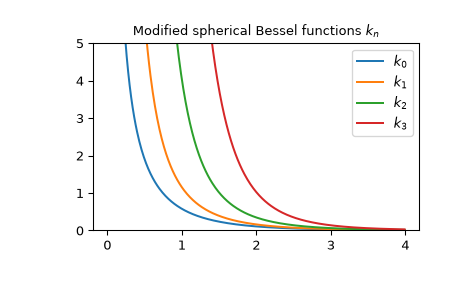# scipy.special.spherical_kn¶

scipy.special.spherical_kn(n, z, derivative=False)[source]

Modified spherical Bessel function of the second kind or its derivative.

Defined as ,

$k_n(z) = \sqrt{\frac{\pi}{2z}} K_{n + 1/2}(z),$

where $$K_n$$ is the modified Bessel function of the second kind.

Parameters
nint, array_like

Order of the Bessel function (n >= 0).

zcomplex or float, array_like

Argument of the Bessel function.

derivativebool, optional

If True, the value of the derivative (rather than the function itself) is returned.

Returns
knndarray

Notes

The function is computed using its definitional relation to the modified cylindrical Bessel function of the second kind.

The derivative is computed using the relations ,

\begin{align}\begin{aligned}k_n' = -k_{n-1} - \frac{n + 1}{z} k_n.\\k_0' = -k_1\end{aligned}\end{align}

New in version 0.18.0.

References

1

https://dlmf.nist.gov/10.47.E9

2

https://dlmf.nist.gov/10.51.E5

AS

Milton Abramowitz and Irene A. Stegun, eds. Handbook of Mathematical Functions with Formulas, Graphs, and Mathematical Tables. New York: Dover, 1972.

Examples

The modified spherical Bessel functions of the second kind $$k_n$$ accept both real and complex second argument. They can return a complex type:

>>> from scipy.special import spherical_kn
>>> spherical_kn(0, 3+5j)
(0.012985785614001561+0.003354691603137546j)
>>> type(spherical_kn(0, 3+5j))
<class 'numpy.complex128'>


We can verify the relation for the derivative from the Notes for $$n=3$$ in the interval $$[1, 2]$$:

>>> from scipy.special import spherical_kn
>>> x = np.arange(1.0, 2.0, 0.01)
>>> np.allclose(spherical_kn(3, x, True),
...             - 4/x * spherical_kn(3, x) - spherical_kn(2, x))
True


The first few $$k_n$$ with real argument:

>>> import matplotlib.pyplot as plt
>>> from scipy.special import spherical_kn
>>> x = np.arange(0.0, 4.0, 0.01)
>>> fig, ax = plt.subplots()
>>> ax.set_ylim(0.0, 5.0)
>>> ax.set_title(r'Modified spherical Bessel functions $k_n$')
>>> for n in np.arange(0, 4):
...     ax.plot(x, spherical_kn(n, x), label=rf'$k_{n}$')
>>> plt.legend(loc='best')
>>> plt.show()